LCTMtools: Latent Class Trajectory Modelling tools: An R Package

Motivation

Latent class trajectory modelling (LCTM) is a relatively new methodology in epidemiology to describe life-course exposures, which simplifies heterogeneous populations into homogeneous patterns or classes. However, for a given dataset, it is possible to derive scores of different models based on number of classes, model structure and trajectory property. To facilitate generalisability of results in future studies, a systematic framework to derive a core favoured model was described in the manuscript “A framework to construct and interpret latent class trajectory modelling” are available in an R package called LCTMtools.

The LCTMtools package provides a quick and easy way to summarise and compare the output of fitted Latent class trajectory models objects. It is primarily aimed at researchers with little experience with R to aid in the analysis of model selection, but we hope may be of use to all.

This vignette illustrates basic use of the package’s function, LCTMtoolkit, for summarising outputs from fitted Latent class trajectory models objects.

To install the R package, in the R console use the command

devtools::install_github(“hlennon/LCTMtools”)

Example

Aim: By modelling BMI as a function of age, identify subgroups of participants with distinct trajectories. We assume an initial $$K=5$$ number of classes of BMI trajectories, based on available literature to date.

To illustrate the functions in the package we use long format data frame of Body Mass Index (BMI) repeated measures of 10,000 individuals, which is included in the LCTMtools package called bmi_long.

An example (simulated) dataset bmi is provided to describe the steps throughout, and bmi_long is the long format version.

Variables included are:

id - Individual ID
age - Age of BMI measure, in years
bmi - Body mass index of the individual at times T1,T2, T3 and T4, in kg/m^2 true_class - Tag to identify the class the individual BMI data was simulated from

library( LCTMtools )
#>
#> Attaching package: 'LCTMtools'
#> The following object is masked _by_ '.GlobalEnv':
#>
#>     gg_color_hue
data( bmi_long, package = "LCTMtools" )

Spaghetti plot the data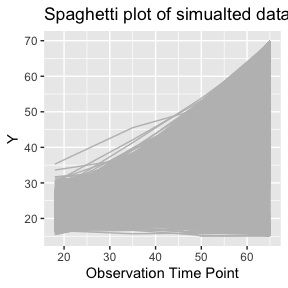An example of the eight step framework for Latent class trajectory modelling

To model longitdinal outcome $$y_{ijk}$$, for $$k=1:K,$$ classes, for individual $$i$$, at time point $$j$$, $$t_j$$ there is are many modelling choices that can be used. We illustrate these here giving the equations, and name them models A to G, in the order of increasing complexity.

Model A: No random effects model | Fixed effects homoscedastic | - with the interpretation that any deviation of an individuals trajectory from its mean class trajectory is due to random error only | Can be fitted in R or in SAS using the PROC TRAJ Macro (B Jones 2007)| (common residual variance across classes)

$y_{ijk}=\beta_0^{(k)} + \beta_1^{(k)} t_{ij} + \beta_2^{(k)} t^2_{ij} + \epsilon_{ij}},$ where the residual variance is assumed equal across all classes, $$\epsilon_{ij}\sim N(0, \sigma^2)$$.

Model B: Fixed effects model with class-specific residual variances | heteroscedastic | The same interpretation as Model A with random errors that can be larger and smaller in different classes. | Can be fit using the R mmlcr package

$y_{ijk}=\beta_0^{(k)} + \beta_1^{(k)} t_{ij} + \beta_2^{(k)} t^2_{ij} + \epsilon_{ijk}},$ where the residual variances are assumed different across $$\epsilon_{ijk} \sim N(0, \sigma_k^2)$$.

Model C: Random intercept The interpretation is allowing individuals to vary in initial weight but each class member is assumed to follow the same shape and magnitude of the mean trajectory SAS traj PROC TRAJ

For $$k=1:K,$$ classes, for individual $$i$$, at time point $$j$$, $$t_j$$, $y_{ijk}=\beta_0^{(k)} + \beta_1^{(k)} t_{ij} + \beta_2^{(k)} t^2_{ij} + b_0^{(k)} + \epsilon_{ij}},$ where the random effect distibruion $$b_0 \sim N(0, B)$$.

Model D: Random slope Allowing individuals to vary in initial weight and slope of the mean trajectory but same curvature as trajectory SAS traj PROC TRAJ For $$k=1:K,$$ classes, for individual $$i$$, at time point $$j$$, $$t_j$$, $y_{ijk}=\beta_0^{(k)} + \beta_1^{(k)} t_{ij} + \beta_2^{(k)} t^2_{ij} + b_0^{(k)} + + b_1^{(k)} t_{ij} \epsilon_{ij}},$ where the random effects assumed to be distributed as $$b_0 \sim N(0, B)$$.

Model E: Random quadratic – Common variance structure across classes Additional freedom of allowing individuals to vary within classes by initial weight, shape and magnitude, however each class is assumed to have the same amount of variability R lcmm hlme/lcmm For $$k=1:K,$$ classes, for individual $$i$$, at time point $$j$$, $$t_j$$, $y_{ijk}=\beta_0^{(k)} + \beta_1^{(k)} t_{ij} + \beta_2^{(k)} t^2_{ij} + b_0^{(k)} + + b_1^{(k)} t_{ij} \epsilon_{ij}},$ where the random effects assumed to be distributed as $$b_0 \sim N(0, B)$$.

Model F and G: Random quadratic – Proportionality constraint to allow variance structures to vary across classes Increasing flexibility of model E as variance structures are allowed to differ up to a multiplicative factor to allow some classes to have larger or smaller within-class variances. This model is can be thought of more parsimonious version of model G from (reducing the number of variance-covariance parameters to be estimated from 6xK parameters to 6+(K-1) parameters. R lcmm hlme/lcmm

For $$k=1:K,$$ classes, for individual $$i$$, at time point $$j$$, $$t_j$$, $y_{ijk}=\beta_0^{(k)} + \beta_1^{(k)} t_{ij} + \beta_2^{(k)} t^2_{ij} + b_0^{(k)} + + b_1^{(k)} t_{ij} \epsilon_{ij}},$ where the random effects assumed to be distributed as $$b_0 \sim N(0, B)$$.

Step 1: Select the form of the random effect structure

To determine the initial working model structure of random effects, the rationale of Verbeke and Molenbergh can be followed to examined the shape of standardised residual plots for each of the $$K$$ classes in a model with no random effects.

If the residual profile could be approximated by a flat, straight line or a curve, then a random intercept, slope or quadratic term, respectively, were considered.

To fit a latent class modelel with no random effects, the lcmm R package this can be used with the specification of $$\verb|random=~-1|$$.

library( lcmm )
model1 <- lcmm::hlme(fixed=bmi~1+age+I(age^2),
mixture = ~1+age+I(age^2),
random=~-1,
subject="id",
ng=5,
nwg=FALSE,
data=data.frame(bmi_long)
)
#> Be patient, hlme is running ...
#> The program took 146.62 seconds

We then feed the fitted model to the step1 function in LCTMtools to examine the class speciifc residuals.

residualplot_step1( model1,
nameofoutcome="bmi",  nameofage = "age",
data = bmi_long,
ylimit=c(-15,15))

STEP 2

Refine the preliminary working model from step 1 to determine the optimal number of classes, testing $$K=1, ... 7$$. The number of classes chosen may be chosen based on the lowest Bayesian information criteria (BIC).

set.seed(100)
m.1 <- lcmm::hlme(fixed = bmi ~ 1+ age + I(age^2),
random = ~ 1 + age,
ng = 1,
idiag = FALSE,
data = data.frame(bmi_long[1:500,]), subject = "id")
#> Be patient, hlme is running ...
#> The program took 0.06 seconds
lin <- c(m.1$ng, m.1$BIC)

for (i in 2:4) {
mi <- lcmm::hlme(fixed = bmi ~ 1+ age + I(age^2),
mixture = ~ 1 + age + I(age^2),
random = ~ 1 + age,
ng = i, nwg = TRUE,
idiag = FALSE,
data = data.frame(bmi_long[1:500,]), subject = "id")

lin <- rbind(lin, c(i, miBIC)) } #> Be patient, hlme is running ... #> The program took 0.29 seconds #> Be patient, hlme is running ... #> The program took 0.69 seconds #> Be patient, hlme is running ... #> The program took 2.3 seconds modelout <- knitr::kable(lin, col.names = c("k", "BIC"), row.names = FALSE, align = "c") modelout k BIC 1 2876.475 2 2688.273 3 2572.130 4 2532.980 Step 3 Further refine the model using the favoured K derived in step 2, testing for the optimal model structure. We tested seven models (detailed above and in the supplementary material Table S2 of the accompanying paper), ranging from a simple fixed effects model (model A) through a rudimentary method that allows the residual variances to vary between classes (model B) to a suite of five random effects models with different variance structures (models C-G). • Model A (SAS, PROC TRAJ)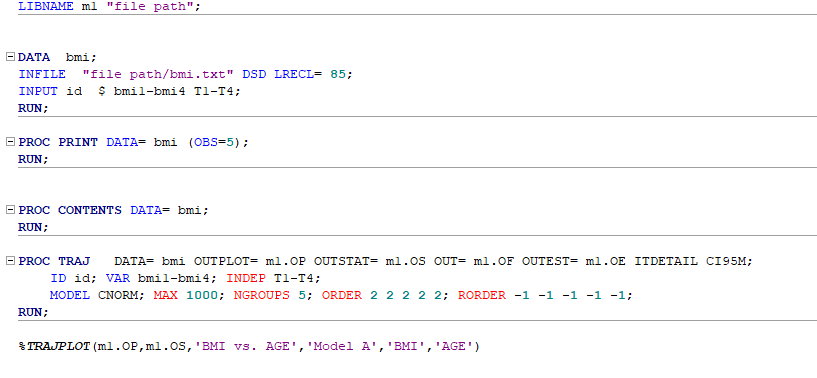oe <- read_sas("oe") # e.g for each model_b <- sastraj_to_lctm(oe, of, op, os) • Model B (R, mmlcr) Use the R package and script from the depreciated R package from https://cran.r-project.org/src/contrib/Archive/mmlcr/ install.packages(“mmlcr_1.3.2.tar.gz”, repos = NULL, type = “source”) or alternatively, save the file “mmlcr.R” into your folder and call the source() command. library( here ) source( here::here("vignettes", "mmlcr.R") ) ls() # model_b <- mmlcr(outer = ~1|id, # components = list(list(formula = bmi ~ 1 + age +I(age^2), # class = "normlong", # min = -1000, # max = 5000)), # data = bmi_long[1:400,], # n.groups = 5, # max.iter = 2000, # tol = 0.001 # ) # model_bBIC

• Model C (SAS, PROC TRAJ)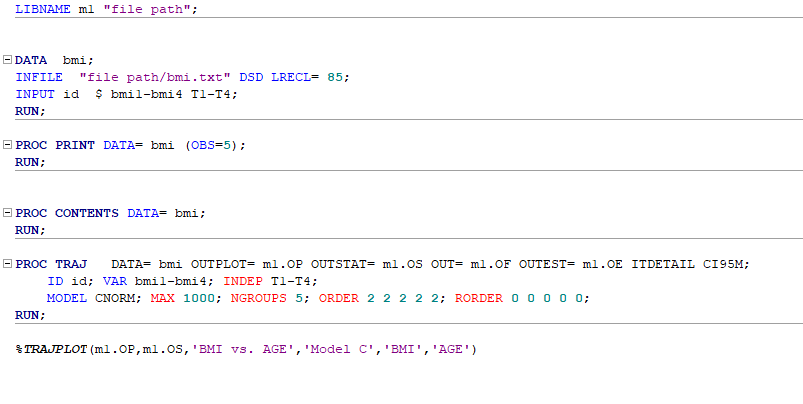• Model D (SAS, PROC TRAJ)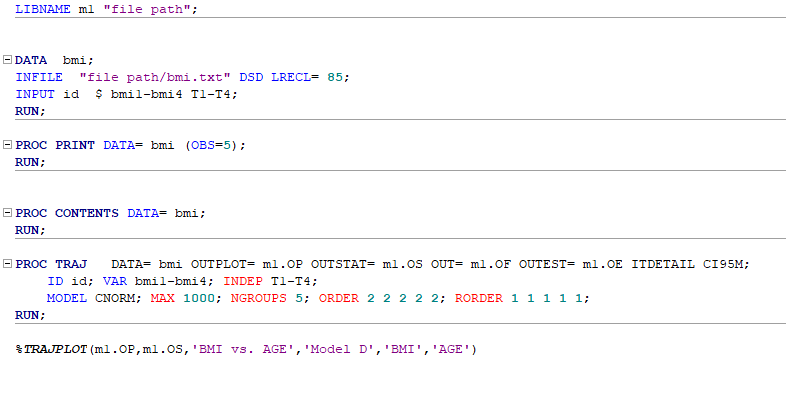• Model E (R, lcmm)
model_e <- hlme(fixed = bmi ~1+ age + I(age^2),
mixture = ~1 + age + I(age^2),
random = ~1 + age,
ng = 5, nwg = F,
idiag = FALSE,
data = data.frame(bmi_long[1:200,]),
subject = "id")
#> Be patient, hlme is running ...
#> The program took 0.77 seconds
model_e$BIC #>  1028.995 • Model F (R, lcmm) model_f <- hlme(fixed = bmi ~1+ age + I(age^2), mixture = ~1 + age + I(age^2), random = ~1 + age, ng = 5, nwg = T, idiag = FALSE, data = data.frame(bmi_long[1:200,]), subject = "id") #> Be patient, hlme is running ... #> The program took 1.58 seconds model_f$BIC
#>  1035.16

• Model G (SAS, PROC TRAJ)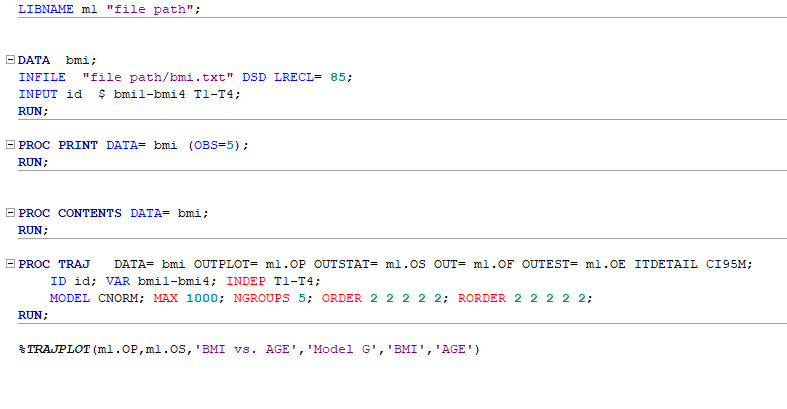Step 4

Perform a number of model adequacy assessments. First, for each participant, calculate the posterior probability of being assigned to each trajectory class and assigned the individual to the class with the highest probability. An average of these maximum posterior probability of assignments (APPA) above 70%, in all classes, is regarded as acceptable. Further assess model adequacy using odds of correct classification, mismatch.

LCTMtoolkit( model_f )
#>  "class(model) type required to be hlme, lcmm or an imported PROC TRAJ object from SAS"
#> $Class-specific #> Class_1 Class_2 Class_3 Class_4 Class_5 Recommendation #> APPA 0.995 0.930 0.889 0.973 0.927 Greater than 0.7 #> OCC 1131.259 65.421 26.225 153.237 38.729 Greater than 5 #> Mismatch 0.000 0.010 0.006 -0.010 -0.006 Close to zero #> #>$Model-specific
#>                     Model Recommendation
#> Entropy             8.298  Close to zero
#> Relative_entropy    0.897     Close to 1
#> BIC              1035.160              -
#> AIC               983.535              -
#> $appa #> Class_1 Class_2 Class_3 Class_4 Class_5 #> APPA 0.995 0.93 0.889 0.973 0.927 #> #>$occ
#>      Class_1 Class_2 Class_3 Class_4 Class_5
#> OCC 1131.259  65.421  26.225 153.237  38.729
#>
#> $mismatch #> Class_1 Class_2 Class_3 Class_4 Class_5 #> Mismatch 0 0.01 0.006 -0.01 -0.006 #> #>$entropy
#>  8.29758
#>
#> $relativeentropy #>  0.8968885 #> #>$BIC
#>  1035.16
#>
#> $AIC #>  983.5353 Step 5 Graphical presentation approaches; 1. Plot mean trajectories with time encompassing each class 2. Mean trajectory plots with 95% predictive intervals for each class, which displays the predicted random variation within each class datnew <- data.frame(age = seq(18, 65, length = 100)) plotpred <- predictY(model_f, datnew, var.time ="age", draws = TRUE) plot(plotpred, lty=1, xlab="Age", ylab="BMI", legend.loc = "topleft", cex=0.75)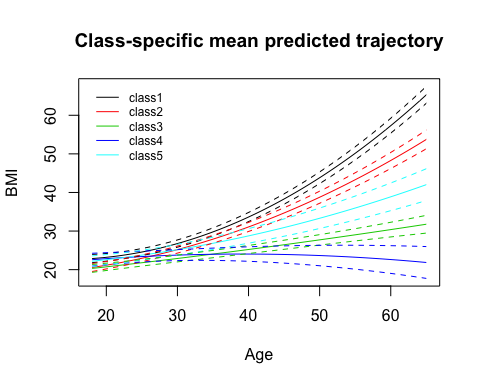1. Individual level ‘spaghetti plots’ with time, depending on sample size maybe use a random sample of participants library(ggplot2) ggplot(bmi_long, aes(x = age, y = bmi)) + geom_line(aes(color = id,group = id), colour = "grey") + xlab("Age") + ylab("BMI")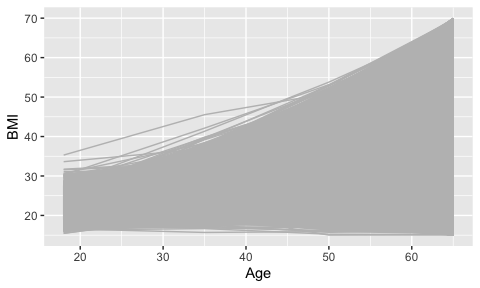ggplot(bmi_long, aes(x = age, y= bmi)) + geom_line(aes(color = true_class, group = id)) + xlab("Age") + ylab("BMI") + labs(color = "Class Assignment")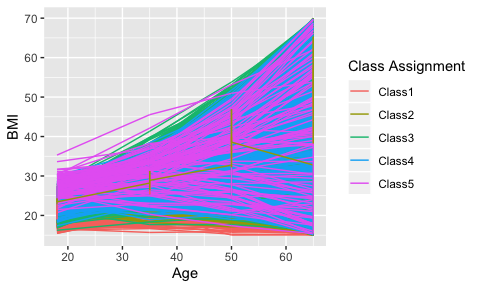Step 6 Assess model discrimination, including degrees of separation ($$DoS_K$$), and Elsensohn’s envelope of residuals. Step 7 Assessing clinical characterisation and plausibility using four approaches; 1. Assessing the clinical meaningfulness of the trajectory patterns, aiming to include classes with at least 1% capture of the population lcmm::postprob( model_f ) #> #> Posterior classification: #> class1 class2 class3 class4 class5 #> N 8 9 12 9 12 #> % 16 18 24 18 24 #> #> Posterior classification table: #> --> mean of posterior probabilities in each class #> prob1 prob2 prob3 prob4 prob5 #> class1 0.9954 0.0046 0.0000 0.0000 0.0000 #> class2 0.0033 0.9305 0.0000 0.0000 0.0662 #> class3 0.0000 0.0000 0.8891 0.0607 0.0502 #> class4 0.0000 0.0000 0.0270 0.9729 0.0001 #> class5 0.0000 0.0067 0.0664 0.0000 0.9268 #> #> Posterior probabilities above a threshold (%): #> class1 class2 class3 class4 class5 #> prob>0.7 100 100.00 83.33 100.00 91.67 #> prob>0.8 100 88.89 83.33 100.00 91.67 #> prob>0.9 100 66.67 66.67 77.78 75.00 #> 2. Assessing the clinical plausibility of the trajectory classes Use the plots generated in 6.2 to assess whether the predicted trends seem realistic for the group that is being studid. E.g. for studying BMI, a predicted trend showing a drop to <5 kg/$$m^2$$ would be unrealistic as this is unsustainable for life. 3. Tabulation of characteristics by latent classes versus conventional categorisations Extract class assignments from chosen model using; model_f$pprob[,1:2]

and then feed back into main dataset with descriptive variables.

Then these can be tabulated as needed.

table(x$class) rbind(by(x$VARIABLE, x$class, meanSD)) e.t.c.. 4. Concordance of class membership with conventional BMI category membership using the kappa statistic # Defining BMI categories, these need to be in equal number to the number of classes derived library(dplyr) library(kableExtra) library(caret) bmi_long <- bmi_long %>% mutate(bmi_class = case_when(bmi<18.5~ 1, bmi>=18.5 & bmi<25 ~ 2, bmi>=25 & bmi<30 ~ 3, bmi>=30 & bmi<35 ~ 4, bmi>=35 ~ 5)) bmi_long$true_class <-  as.factor(bmi_long$true_class) bmi_long$bmi_class <- as.factor(bmi_long$bmi_class) levels(bmi_long$true_class) <- c("1","2","3", "4", "5")
x <- broom::confusionMatrix(bmi_long$true_class, bmi_long$bmi_class, dnn=c("Latent Class", "BMI Class"))
y <- as.matrix(x\$table)
colnames(y) <- c("<18.5", "18.5-24.9", "25 - 29.9", "30.0-34.9", "<35")
kable(y, row.names = T, align="c") %>%
column_spec(1, bold = T, border_right = T) %>%
kable_styling() %>%